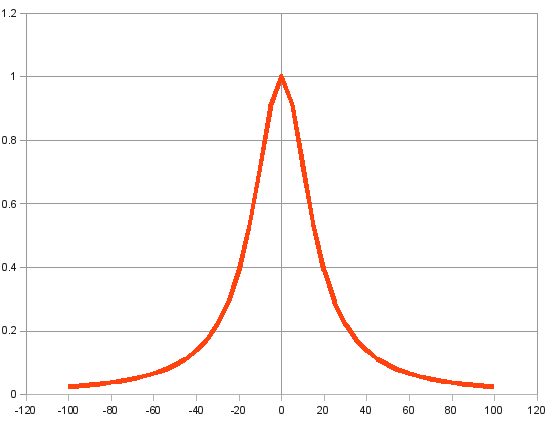### The Witch of Agnesi

June 22, 2010

The science fiction author and biochemist, Isaac Asimov had a simple test of whether a person was a scientist or a non-scientist. He would ask them to pronounce the word, "unionized." If they pronounced it with an initial you sound, as the word for a worker's collective organization, they were non-scientists. If they pronounced it with an initial un sound, as un-ion-ized, then they were scientists. I have a similar test for mathematicians, and this test is made all the easier by the Harry Potter books. If a person thinks the "Witch of Agnesi" is a character from that sort of book, then he's not a mathematician. Mathematicians know that the Witch of Agnesi is a mathematical function.

The Witch of Agnesi is a mathematical function first studied by Pierre de Fermat in 1630. It was popularized in 1748 by Maria Agnesi, the daughter of an wealthy Italian silk merchant. Agnesi published it in her two-volume treatise on mathematics, "Instituzioni analitiche," that contained more than a thousand pages.  The first volume concerned arithmetic, geometry, trigonometry, analytical geometry and calculus, while the second volume contained infinite series and differential equations.  The function is interesting because it can be constructed geometrically . It is written, as follows:

y = 8a3/(4a2 + x2)

where a is a constant. For a = 1/2, this becomes

y = 1/(1 + x2)

The figure below illustrates the form of the curve.The Witch of Agnesi with parameter a=8, normalized to peak at y=1.

How did this function get its name? Agnesi called it la versiera. This is a nautical term for a rope that turns a sail , an allusion to the curve's geometrical construction. When her book was translated into English by John Colson, a Cambridge professor, he apparently misread the word; or his knowledge of Italian did not include such an obscure nautical term. He read it as l'avversiera, meaning "witch."

The Witch of Agnesia is a useful function, since it can replace the Normal Distribution in some mathematical models, and it can be easily integrated. As can be inferred from its simplicity, it can describe a number of physical systems, such as a driven oscillator near resonance. 

Agnesi was the first woman appointed as a mathematics professor at a university; in that case, the University of Bologna, but it doesn't appear that she ever taught there. She left mathematics upon the death of her father and became a nun, founding homes for the poor, ill and elderly. 

Isaac Asimov's book are cataloged in nine of the ten major categories of the Dewey Decimal Classification. He never wrote a book in the "Philosophy and Psychology" category. I would think, for a scientist, that would be the easiest category in which to publish.